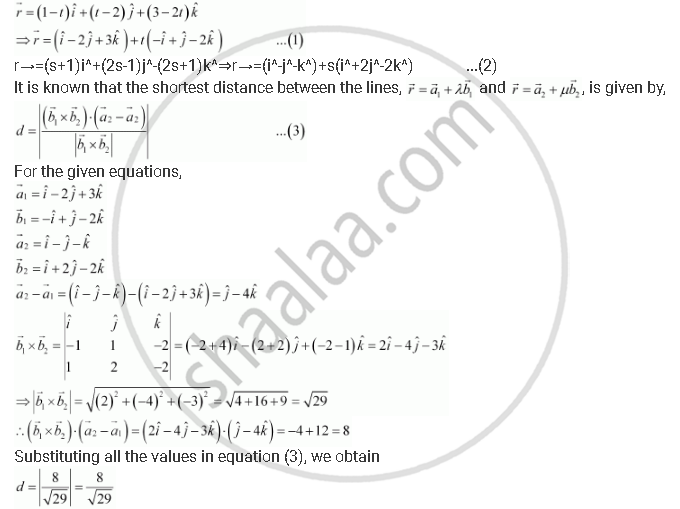Share

# Find the Shortest Distance Between the Lines Whose Vector Equations Are Vecr = (1-t)Hati + (T - 2)Hatj + (3 -2t)Hatk and Vecr = (S+1)Hati + (2s + 1)Hatk - CBSE (Commerce) Class 12 - Mathematics

ConceptShortest Distance Between Two Lines

#### Question

Find the shortest distance between the lines whose vector equations are

vecr = (1-t)hati + (t - 2)hatj + (3 -2t)hatk and vecr = (s+1)hati + (2s + 1)hatk

#### Solution

The given lines areTherefore, the shortest distance between the lines is 8/sqrt29 units.

Is there an error in this question or solution?

#### APPEARS IN

NCERT Solution for Mathematics Textbook for Class 12 (2018 to Current)
Chapter 11: Three Dimensional Geometry
Q: 17 | Page no. 478

#### Video TutorialsVIEW ALL 

Solution Find the Shortest Distance Between the Lines Whose Vector Equations Are Vecr = (1-t)Hati + (T - 2)Hatj + (3 -2t)Hatk and Vecr = (S+1)Hati + (2s + 1)Hatk Concept: Shortest Distance Between Two Lines.
S